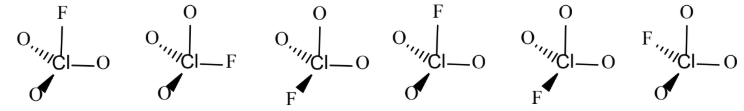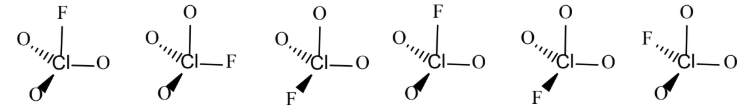Chemistry Practice Problems Boltzmann Equation Practice Problems Solution: FClO3 is a tetrahedral molecule with chlorine (Cl)...

# Solution: FClO3 is a tetrahedral molecule with chlorine (Cl) at the center. When FClO 3 crystallizes in the solid state, there is no preference for one orientation over another. In other words, the unique F atom ends up randomly distributed among the tetrahedral sites, as shown below.Calculate the expected residual molar entropy S for FClO 3 at 0 K. Show all work and circle your final answer.A. 2.47x10-23 J/mol•KB. 14.89 J/mol•KC. 1.91x10-23 J/mol•KD. 11.52 J/mol•KE. 0 J/mol•K

###### Problem

FClO3 is a tetrahedral molecule with chlorine (Cl) at the center. When FClO 3 crystallizes in the solid state, there is no preference for one orientation over another. In other words, the unique F atom ends up randomly distributed among the tetrahedral sites, as shown below.Calculate the expected residual molar entropy S for FClO 3 at 0 K. Show all work and circle your final answer.

A. 2.47x10-23 J/mol•K

B. 14.89 J/mol•K

C. 1.91x10-23 J/mol•K

D. 11.52 J/mol•K

E. 0 J/mol•K

###### Solution

We’re being asked to calculate the expected residual molar entropy (S) for FClO3 at 0.

The possible ways for FClO3 to arrange itself and the unique F atom ends up randomly distributed among the tetrahedral sites, as shown below:View Complete Written Solution

Boltzmann Equation

Boltzmann Equation

#### Q. Consider a system that consists of two standard playing dice, with the state of the system defined by the sum of the values shown on the top faces.The...

Solved • Thu Jan 03 2019 09:45:11 GMT-0500 (EST)

Boltzmann Equation

#### Q. Consider a system that consists of two standard playing dice, with the state of the system defined by the sum of the values shown on the top faces.Cal...

Solved • Thu Jan 03 2019 09:45:11 GMT-0500 (EST)

Boltzmann Equation

#### Q. Indicate whether each statement is true or false.CO2(g) and Ar(g) have nearly the same molar mass. At a given temperature, they will have the same num...

Solved • Thu Jan 03 2019 09:45:10 GMT-0500 (EST)

Boltzmann Equation

#### Q. Two systems, each composed of three particles represented by circles, have 30 J of total energy.How many energetically equivalent ways can you distrib...

Solved • Thu Jan 03 2019 09:31:08 GMT-0500 (EST)